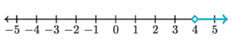Mathematics
Easy

Question

# Identify the inequality that matches the picture.## x < 4    x > 4    x ≥ 4    x ≤ 5Hint:

## The correct answer is: x > 4

### The blue line on the graph represents the inequality. It starts at 4 and continues further.Let x denotes the values satisfying the inequality.So, the variable satisfying the inequality is greater than 4.Now, we have to check if the values also included 4. From the graph, at point 4 there is a open circle. So, 4 doesn’t satisfy the inequality. It’s not included in the given values.The variable x is greater than 4 is the inequality.Mathematical form: x > 4

We have to be careful about the limits of the inequality to solve the question.

### Related Questions to study#### With Turito Foundation.#### Get an Expert Advice From Turito.